# NCERT Solutions For Class 10 Maths Chapter 8 Exercise 8.2 – Introduction to Trignometry

Download NCERT Solutions For Class 10 Maths Chapter 8 Exercise 8.2 – Introduction to Trignometry. This Exercise contains 4 questions, for which detailed answers have been provided in this note. In case you are looking at studying the remaining Exercise for Class 10 for Maths NCERT solutions for other Chapters, you can click the link at the end of this Note.

### NCERT Solutions For Class 10 Maths Chapter 8 Exercise 8.2 – Introduction to Trignometry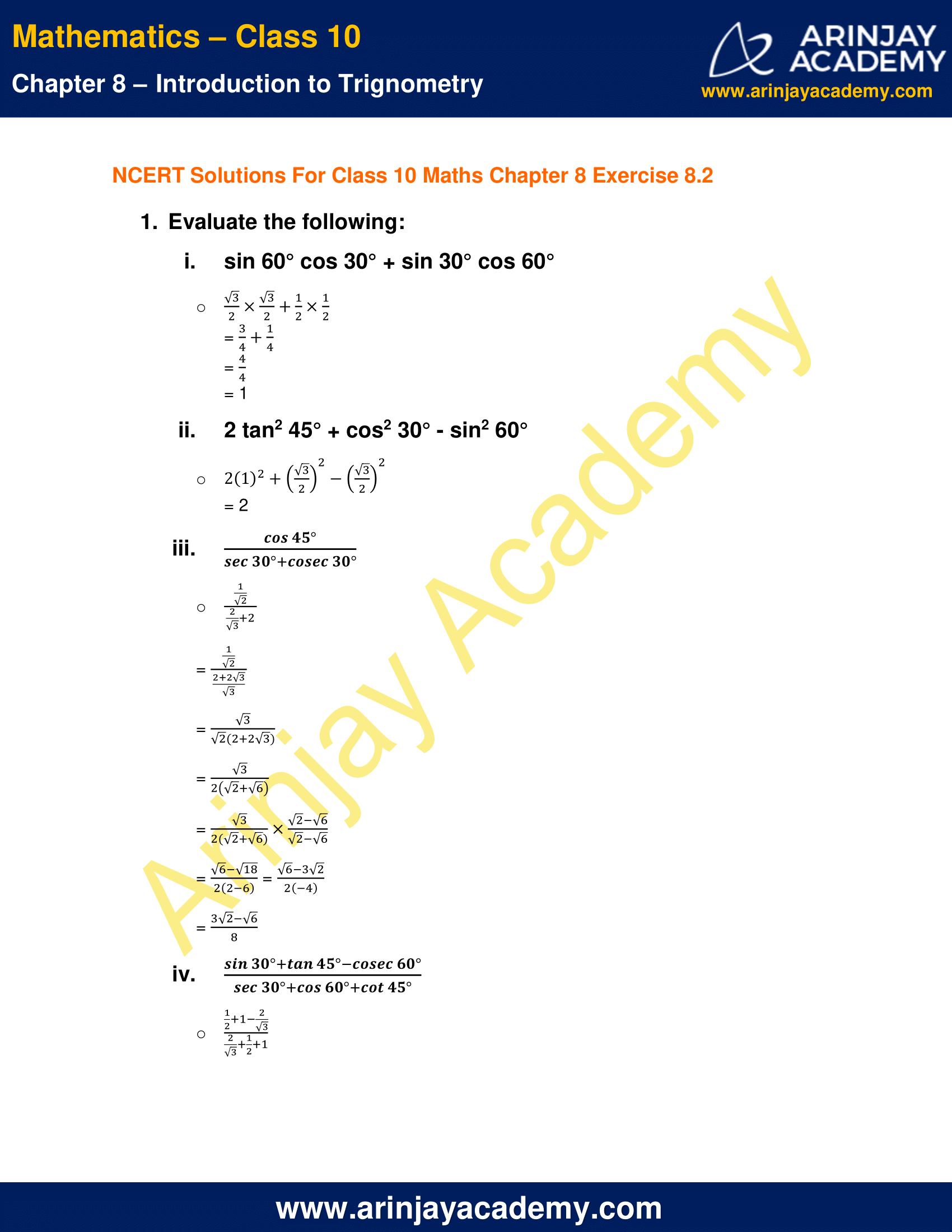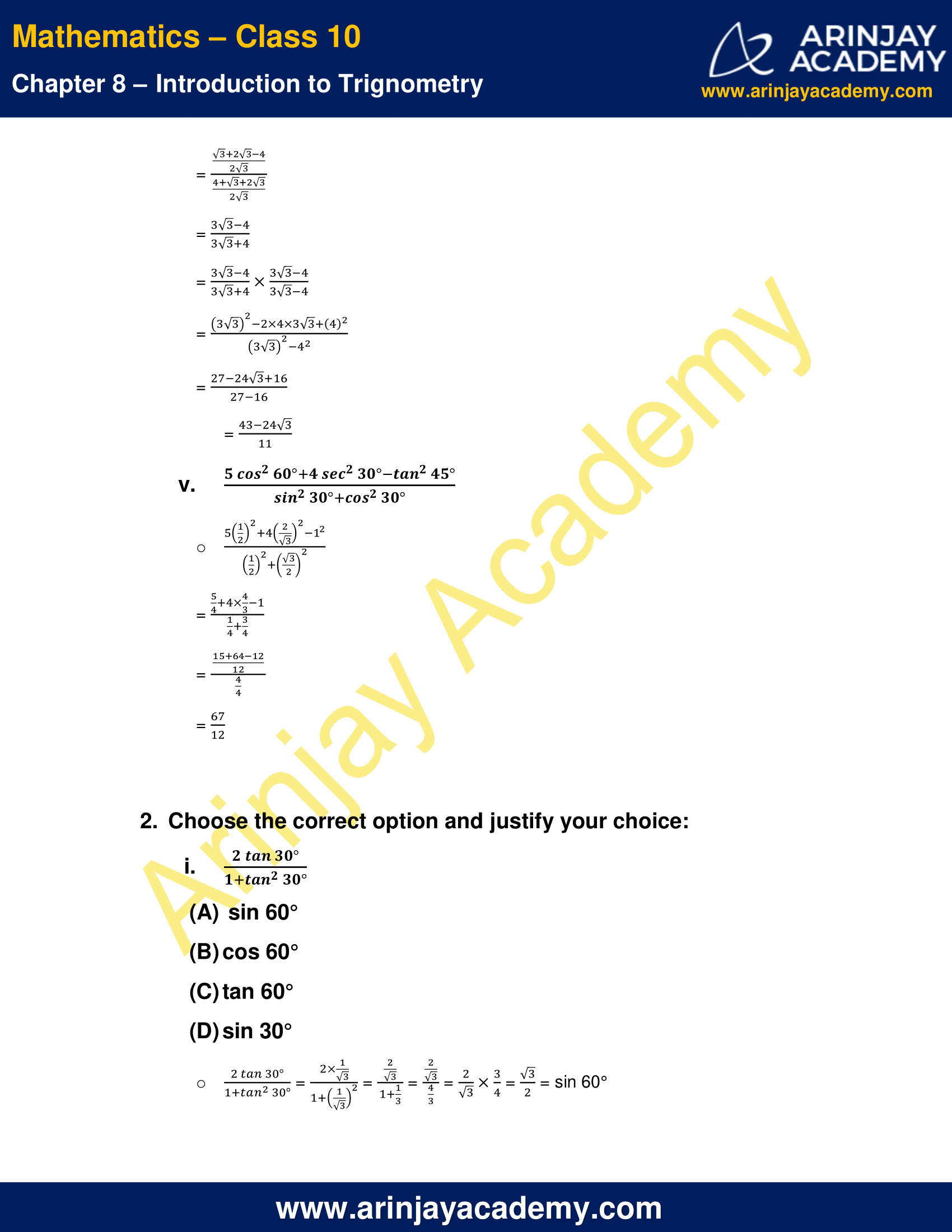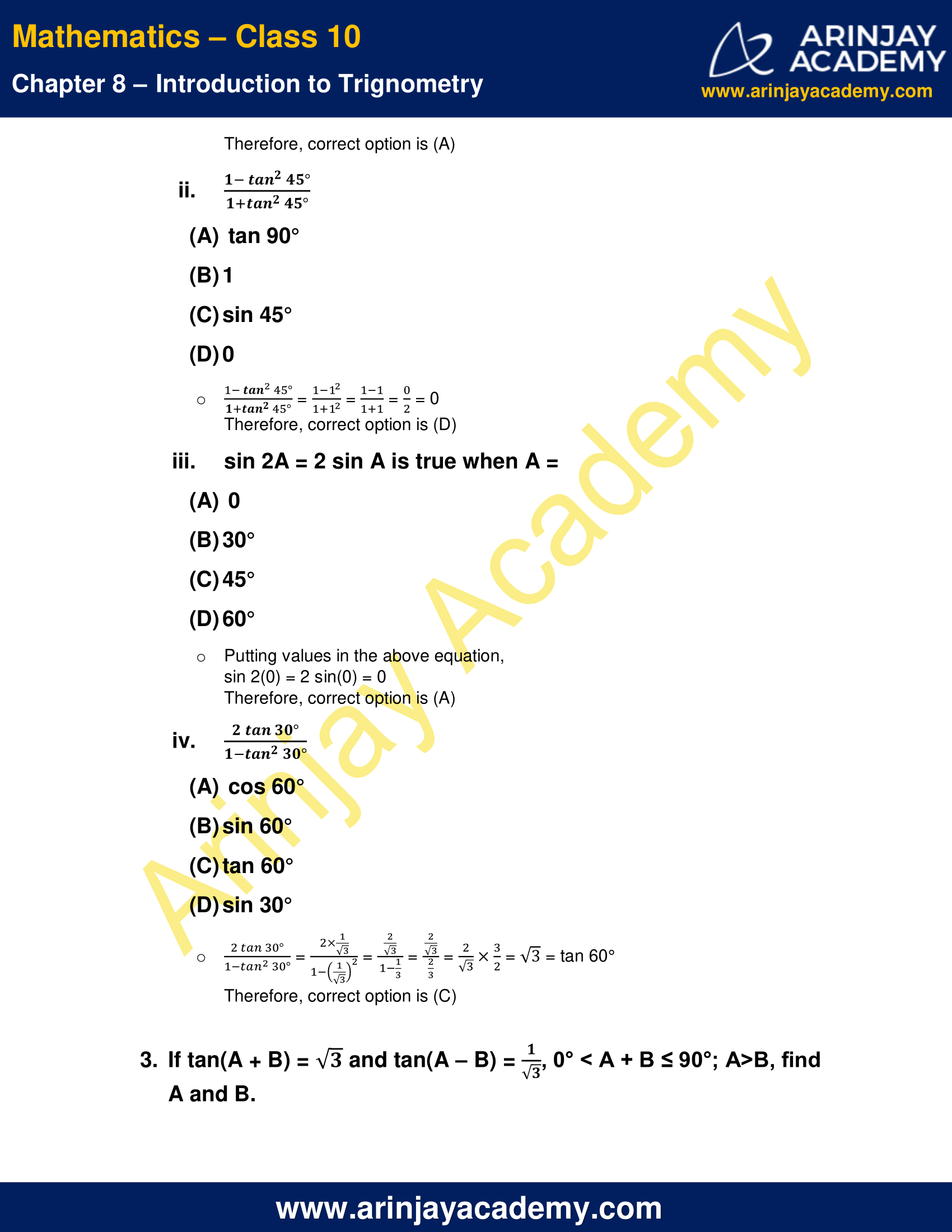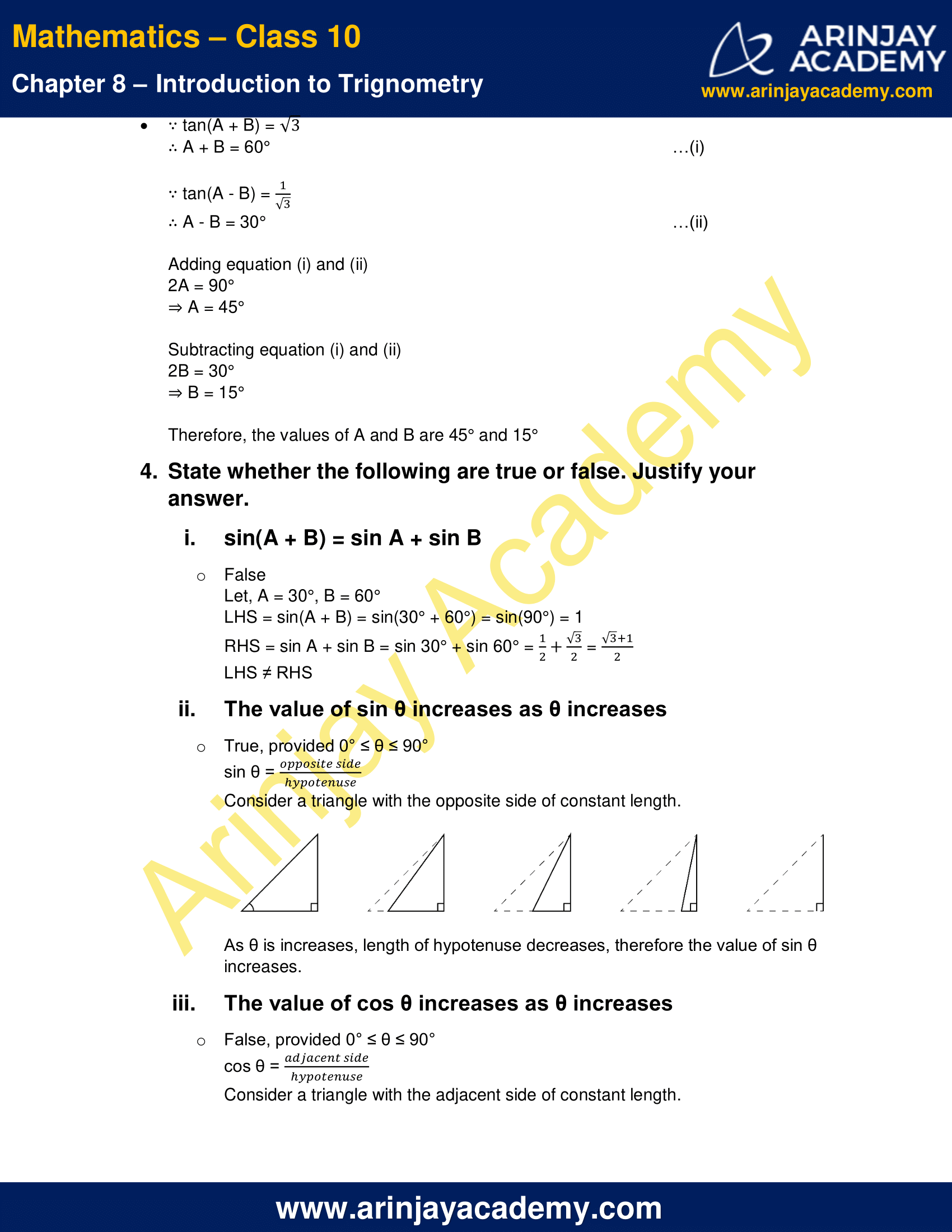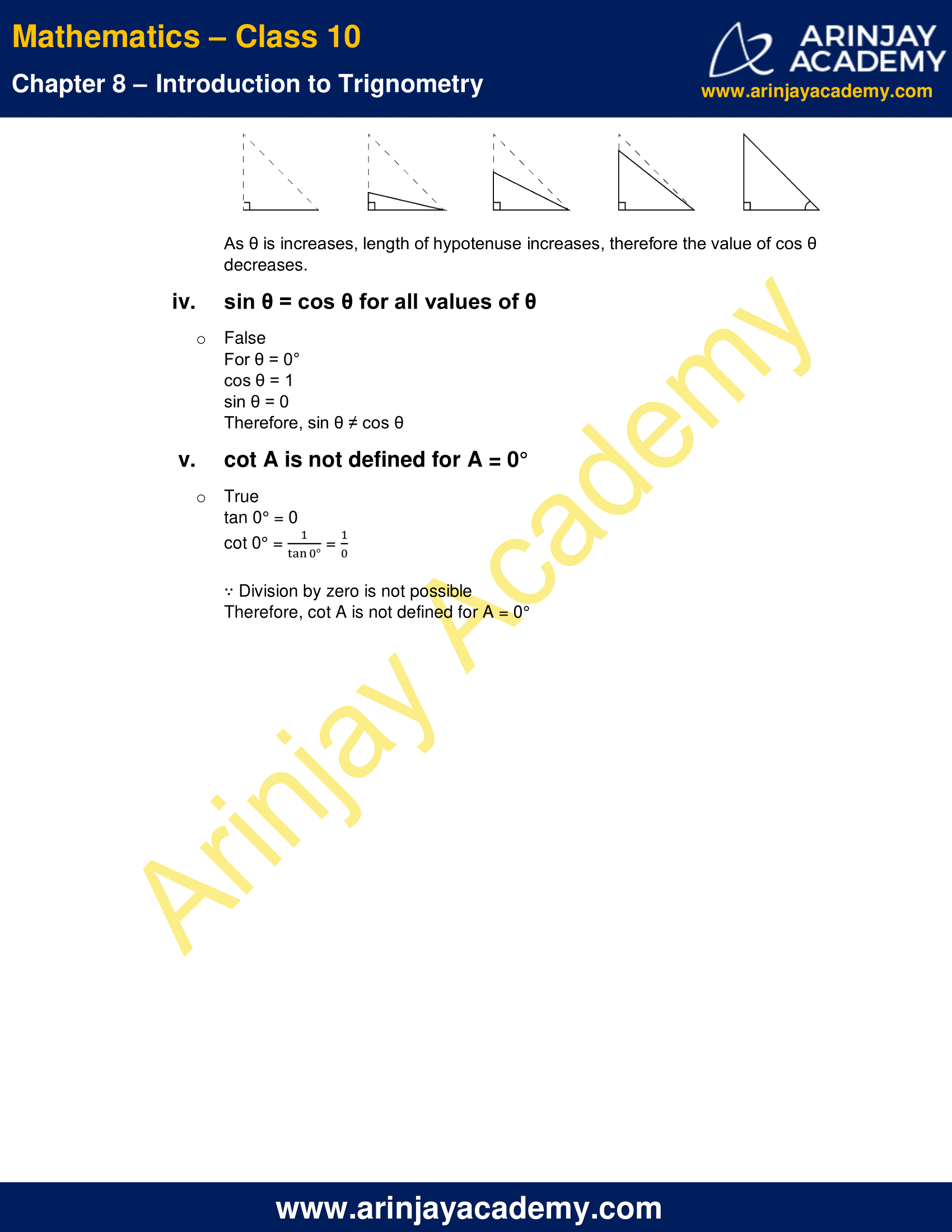NCERT Solutions For Class 10 Maths Chapter 8 Exercise 8.2 – Introduction to Trignometry

1. Evaluate the following:

i. sin 60° cos 30° + sin 30° cos 60°

=$\cfrac { \sqrt { 3 } }{ 2 }$ x$\cfrac { \sqrt { 3 } }{ 2 }$ +$\cfrac { 1 }{ 2 }$ x$\cfrac { 1 }{ 2 }$
=$\cfrac { 3 }{ 4 }$ +$\cfrac { 1 }{ 4 }$
=$\cfrac { 4 }{ 4 }$
= 1

ii. 2 tan2 45° + cos2 30° – sin2 60°$2{ (1) }^{ 2 }+{ \left( \cfrac { \sqrt { 3 } }{ 2 } \right) }^{ 2 }-{ \left( \cfrac { \sqrt { 3 } }{ 2 } \right) }^{ 2 }$
= 2

iii.$\cfrac { cos{ 45 }^{ \circ } }{ { sec }30^{ \circ }+cosec{ 30 }^{ \circ } }$

=$\cfrac { \cfrac { 1 }{ \sqrt { 2 } } }{ \cfrac { 2 }{ \sqrt { 3 } } +2 }$
=$\cfrac { \cfrac { 1 }{ \sqrt { 2 } } }{ \cfrac { 2+2\sqrt { 3 } }{ \sqrt { 3 } } }$
=$\cfrac { \sqrt { 3 } }{ \sqrt { 2 } (2+2\sqrt { 3 } ) }$
=$\cfrac { \sqrt { 3 } }{ 2(\sqrt { 2 } +\sqrt { 6 } ) }$
=$\cfrac { \sqrt { 3 } }{ 2(\sqrt { 2 } +\sqrt { 6 } ) } \times \cfrac { \sqrt { 2 } -\sqrt { 6 } }{ \sqrt { 2 } -\sqrt { 6 } }$
=$\cfrac { \sqrt { 6 } -\sqrt { 18 } }{ 2(2-6) }$
=$\cfrac { \sqrt { 6 } -3\sqrt { 2 } }{ 2(-4) }$$\cfrac { 3\sqrt { 2 } -\sqrt { 6 } }{ 8 }$

iv.$\cfrac { { sin30 }^{ \circ }+{ tan45 }^{ \circ }-cosec{ 60 }^{ \circ } }{ { sec30 }^{ \circ }+{ cos60 }^{ \circ }+cot45^{ \circ } }$

=$\cfrac { \cfrac { 1 }{ 2 } +1-\cfrac { 2 }{ \sqrt { 3 } } }{ \cfrac { 2 }{ \sqrt { 3 } } +\cfrac { 1 }{ 2 } +1 }$
=$\cfrac { \cfrac { \sqrt { 3 } +2\sqrt { 3 } -4 }{ 2\sqrt { 3 } } }{ \cfrac { 4+\sqrt { 3 } +2\sqrt { 3 } }{ 2\sqrt { 3 } } }$
=$\cfrac { 3\sqrt { 3 } -4 }{ 3\sqrt { 3 } +4 }$
=$\cfrac { 3\sqrt { 3 } -4 }{ 3\sqrt { 3 } +4 } \times \cfrac { 3\sqrt { 3 } -4 }{ 3\sqrt { 3 } -4 }$
=$\cfrac { \left( { 3\sqrt { 3 } } \right) ^{ 2 }-2\times 4\times { 3\sqrt { 3 } }+{ 4 }^{ 2 } }{ \left( { 3\sqrt { 3 } } \right) ^{ 2 }-{ 4 }^{ 2 } }$
=$\cfrac { 27-24{ \sqrt { 3 } }+16 }{ 27-16 }$
=$\cfrac { 43-24{ \sqrt { 3 } } }{ 11 }$

v.$\cfrac { 5{ cos }^{ 2 }{ 60 }^{ \circ }+4sec^{ 2 }{ 30 }^{ \circ }-tan^{ 2 }{ 45 }^{ \circ } }{ sin^{ 2 }{ 30 }^{ \circ }+{ cos }^{ 2 }{ 30 }^{ \circ } }$

=$\cfrac { 5{ \left( \cfrac { 1 }{ 2 } \right) }^{ 2 }+4{ \left( \cfrac { 2 }{ \sqrt { 3 } } \right) }^{ 2 }-1^{ 2 } }{ { \left( \cfrac { 1 }{ 2 } \right) }^{ 2 }+{ \left( \cfrac { \sqrt { 3 } }{ 2 } \right) }^{ 2 } }$
=$\cfrac { \cfrac { 5 }{ 4 } +4\times \cfrac { 4 }{ 3 } -1 }{ \cfrac { 1 }{ 4 } +\cfrac { 3 }{ 4 } }$
=$\cfrac { \cfrac { 15+64-12 }{ 12 } }{ \cfrac { 4 }{ 4 } }$
=$\cfrac { 67 }{ 12 }$

2. Choose the correct option and justify your choice:

i.$\cfrac { 2tan{ 30 }^{ \circ } }{ 1+{ tan }^{ 2 }{ 30 }^{ \circ } }$

(A) sin 60°
(B) cos 60°
(C) tan 60°
(D) sin 30°$\cfrac { 2tan{ 30 }^{ \circ } }{ 1+{ tan }^{ 2 }{ 30 }^{ \circ } }$
=$\cfrac { 2\times \cfrac { 1 }{ \sqrt { 3 } } }{ 1+{ \left( \cfrac { 1 }{ \sqrt { 3 } } \right) }^{ 2 } }$
=$\cfrac { \cfrac { 2 }{ \sqrt { 3 } } }{ 1+{ \cfrac { 1 }{ 3 } } }$
=$\cfrac { 2 }{ \sqrt { 3 } } \times \cfrac { 3 }{ 4 }$
=$\cfrac { \sqrt { 3 } }{ 2 }$
= sin 60°
Therefore, correct option is (A)

ii.$\cfrac { 1-{ tan }^{ 2 }{ 45 }^{ \circ } }{ 1{ +tan }^{ 2 }{ 45 }^{ \circ } }$

(A) tan 90°
(B) 1
(C) sin 45°
(D) 0$\cfrac { 1-{ tan }^{ 2 }{ 45 }^{ \circ } }{ 1{ +tan }^{ 2 }{ 45 }^{ \circ } }$
=$\cfrac { 1-1^{ 2 } }{ 1+1^{ 2 } }$
=$\cfrac { 1-1 }{ 1+1 }$
=$\cfrac { 0 }{ 2 }$
= 0
Therefore, correct option is (D)

iii. sin 2A = 2 sin A is true when A =

(A) 0
(B) 30°
(C) 45°
(D) 60°

Putting values in the above equation,
sin 2(0) = 2 sin(0) = 0
Therefore, correct option is (A)

iv.$\cfrac { 2tan{ 30 }^{ \circ } }{ 1-{ tan }^{ 2 }{ 30 }^{ \circ } }$

(A) cos 60°
(B) sin 60°
(C) tan 60°
(D) sin 30°$\cfrac { 2tan{ 30 }^{ \circ } }{ 1-{ tan }^{ 2 }{ 30 }^{ \circ } }$
=$\cfrac { 2\times \cfrac { 1 }{ \sqrt { 3 } } }{ 1{ -\left( \cfrac { 1 }{ \sqrt { 3 } } \right) }^{ 2 } }$
=$\cfrac { \cfrac { 2 }{ \sqrt { 3 } } }{ 1-{ \cfrac { 1 }{ 3 } } }$
=$\cfrac { \cfrac { 2 }{ \sqrt { 3 } } }{ { \cfrac { 2 }{ 3 } } }$
=$\cfrac { 2 }{ \sqrt { 3 } } \times \cfrac { 3 }{ 2 }$=$\sqrt { 3 }$
= tan 60°
Therefore, correct option is (C)

3. If tan(A + B) = √3 and tan(A – B) =$\cfrac { 1 }{ \sqrt { 3 } }$, 0° < A + B ≤ 90°; A>B, find A and B.

∵ tan(A + B) = √3
∴ A + B = 60°    …(i)
∵ tan(A – B) =$\cfrac { 1 }{ \sqrt { 3 } }$
∴ A – B = 30°     …(ii)
2A = 90°
⇒ A = 45°
Subtracting equation (i) and (ii)
2B = 30°
⇒ B = 15°
Therefore, the values of A and B are 45° and 15°

4. State whether the following are true or false. Justify your answer.

i. sin(A + B) = sin A + sin B

False
Let, A = 30°, B = 60°
LHS = sin(A + B) = sin(30° + 60°)
= sin(90°)
= 1
RHS = sin A + sin B = sin 30° + sin 60°
=$\cfrac { 1 }{ 2 }$ +$\cfrac { \sqrt { 3 } }{ 2 }$
=$\cfrac { \sqrt { 3 } +1 }{ 2 }$
LHS ≠ RHS

ii. The value of sin θ increases as θ increases

True, provided 0° ≤ θ ≤ 90°
sin θ =$\cfrac { opposite\quad side }{ hypotenuse }$
Consider a triangle with the opposite side of constant length.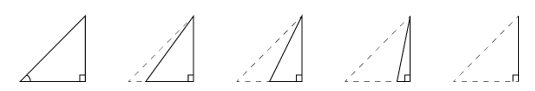As θ is increases, length of hypotenuse decreases, therefore the value of sin θ increases.

iii. The value of cos θ increases as θ increases

False, provided 0° ≤ θ ≤ 90°
cos θ =$\cfrac { adjacent\quad side }{ hypotenuse }$
Consider a triangle with the adjacent side of constant length.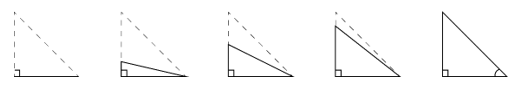As θ is increases, length of hypotenuse increases, therefore the value of cos θ decreases.

iv. sin θ = cos θ for all values of θ

False
For θ = 0°
cos θ = 1
sin θ = 0
Therefore, sin θ ≠ cos θ

v. cot A is not defined for A = 0°

True
tan 0° = 0
cot 0° =$\cfrac { 1 }{ { tan0 }^{ \circ } }$ =$\cfrac { 1 }{ 0 }$
∵ Division by zero is not possible
Therefore, cot A is not defined for A = 0°

NCERT Solutions for Class 10 Maths Chapter 8 Exercise 8.2- Introduction to Trignometry, has been designed by the NCERT to test the knowledge of the student on the topic – Trigonometric Ratios of Some Specific Angles

Download NCERT Solutions For Class 10 Maths Chapter 8 Exercise 8.2 – Introduction to Trignometry